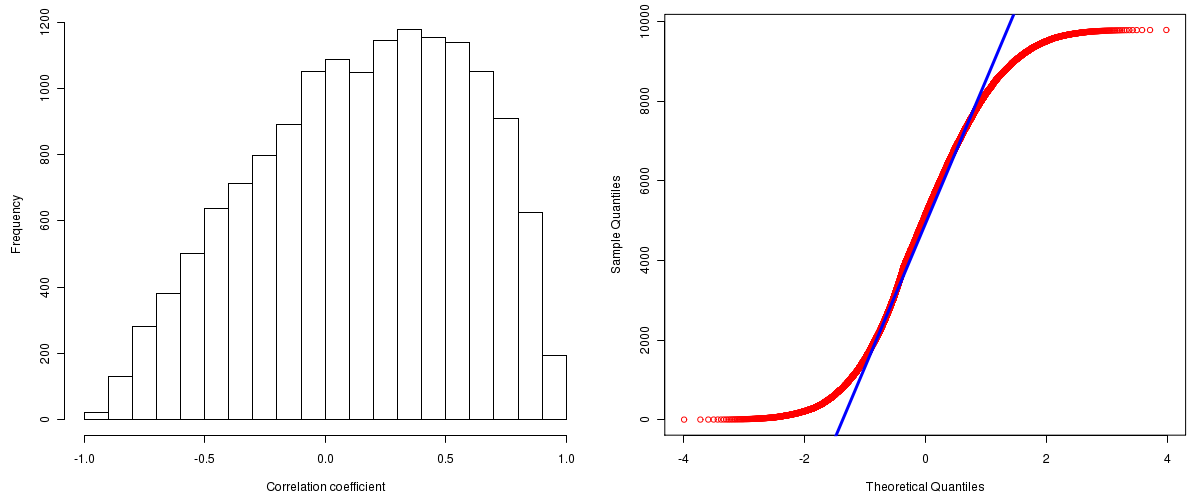Kidney Renal Papillary Cell Carcinoma: Correlations between copy number and mRNA expression
Maintained by John Zhang (Dana-Farber Cancer Institute)
Overview
Introduction

A TCGA sample is profiled to detect the copy number variations and expressions of genes. This pipeline attempts to correlate copy number and expression data of genes across samples to determine if the copy number variations also result in differential expressions. This report contains the calculated correlation coefficients based on measurements of genomic copy number (log2) values and intensity of the expressions of the corresponding feature across patients. High positive/low negative correlation coefficients indicate that genomic alterations result in differences in the expressions of mRNA the genomic regions transcribe.

Summary

The correlation coefficients in 10, 20, 30, 40, 50, 60, 70, 80, 90 percentiles are -0.47075, -0.2572, -0.0865, 0.0538, 0.19315, 0.3214, 0.4517, 0.581, 0.7221, respectively.

Results
Correlation results

Number of genes and samples used for the calculation are shown in Table 1. Figure 1 shows the distribution of calculated correlation coefficients and quantile-quantile plot of the calculated correlation coefficients against a normal distribution. Table 2 shows the top 20 features ordered by the value of correlation coefficients.

Table 1.  Counts of mRNA and number of samples in copy number and expression data sets and common to both

Category Copy number Expression Common
Sample 43 16 8
Genes 29390 17815 15551

Figure 1.  Summary figures. Left: histogram showing the distribution of the calculated correlations across samples for all Genes. Right: QQ plot of the calculated correlations across samples. The QQ plot is used to plot the quantiles of the calculated correlation coefficients against that derived from a normal distribution. Points deviating from the blue line indicate deviation from normality.Table 2.  Get Full Table Top 20 features (defined by the feature column) ranked by correlation coefficients

feature r p-value q-value chrom start end geneid
C6orf162 0.9908 1.90988421922e-06 0.0216761689832592 6 88103446 88108196 57150
KIAA1586 0.9873 5.08605225713055e-06 0.0288619925422163 6 57019343 57027954 57691
IFNW1 0.9846 0.00228188174867383 0.121283164050005 9 21130631 21132144 3467
CCDC23 0.9835 1.09973555733056e-05 0.0368484678660656 1 43045320 43055510 374969
CTAGE5 0.9826 1.29868534120003e-05 0.0368484678660656 14 38804247 38890342 4253
SQSTM1 0.9803 1.88506140199607e-05 0.0368911343805544 5 179180503 179197684 8878
OTOS 0.9793 2.19700645918142e-05 0.0368911343805544 2 240727119 240728746 150677
CDK6 0.9776 2.74532308095488e-05 0.0368911343805544 7 92072171 92301148 1021
FER1L3 0.9776 2.76697204075749e-05 0.0368911343805544 10 95056176 95232029 26509
YRDC 0.9771 2.95960677991758e-05 0.0368911343805544 1 38041201 38046452 79693
ACADVL 0.9763 3.25047269362777e-05 0.0368911343805544 17 7063877 7069309 37
ATR 0.9741 4.26365814143459e-05 0.0382720559127756 3 143650769 143780341 545
STARD4 0.9734 4.62811089787518e-05 0.0382720559127756 5 110861921 110876056 134429
DDX24 0.9732 4.72100369552919e-05 0.0382720559127756 14 93587021 93617311 57062
MTBP 0.9703 6.40410364467492e-05 0.0444302610445739 8 121526847 121605056 27085
DKFZp434B1231 0.97 6.60081604937357e-05 0.0444302610445739 1 199448393 199464697 91156
ZNF124 0.9699 6.65506495329815e-05 0.0444302610445739 1 245385826 245401941 7678
C1orf26 0.9673 8.51691528573895e-05 0.0537013631774488 1 183392914 183527443 54823
POMGNT1 0.9643 0.000110765950121383 0.0614619222538718 1 46426942 46436685 55624
PRKAA2 0.9637 0.0001164957521822 0.0614619222538718 1 56883608 56946709 5563
Methods & Data
Input

Gene level (TCGA Level III) expression data and copy number data of the corresponding loci derived by using the CNTools package of Bioconductor were used for the calculations. Pearson correlation coefficients were calculated for each pair of genes shared by the two data sets across all the samples that were common.

Correlation across sample

Pairwise correlations between the log2 copy numbers and expressions of each gene across samples were calculated using Pearson correlation.